1. /
2. CBSE
3. /
4. Class 12
5. /
6. Physics
7. /
8. NCERT Solutions class 12...

# NCERT Solutions class 12 physics Electrostatic Potentia and Capacitance Part 2### myCBSEguide App

Download the app to get CBSE Sample Papers 2023-24, NCERT Solutions (Revised), Most Important Questions, Previous Year Question Bank, Mock Tests, and Detailed Notes.

Download NCERT solutions for Electrostatic Potentia and Capacitance Part 2  as PDF.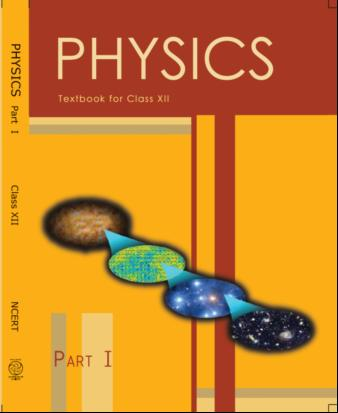## NCERT Class 12 Physics Chapter-wise Solutions

• 1 – Electric Charges and Fields
• 2 – Electrostatic Potential and Capacitance
• 3 – Current Electricity
• 4 – Moving Charges and Magnetism
• 5 – Magnetism and Matter
• 6 – Electromagnetic Induction
• 7 – Alternating Current
• 8 – Electromagnetic Waves
• 9 – Ray Optics and Optical Instruments
• 10 – Wave Optics
• 11 – Dual Nature of Radiation and Matter
• 12 – Atoms
• 13 – Nuclei
• 14 – Semiconductor Electronic: Material, Devices and Simple Circuits
• 15 – Communication Systems

## CHAPTER 2 ELECTROSTATIC POTENTIAL AND CAPACITANCE

• 2.1 Introduction
• 2.2 Electrostatic Potential
• 2.3 Potential due to a Point Charge
• 2.4 Potential due to an Electric Dipole
• 2.5 Potential due to a System of Charges
• 2.6 Equipotential Surfaces
• 2.7 Potential Energy of a System of Charges
• 2.8 Potential Energy in an External Field
• 2.9 Electrostatics of Conductors
• 2.10 Dielectrics and Polarisation
• 2.11 Capacitors and Capacitance
• 2.12 The Parallel Plate Capacitor
• 2.13 Effect of Dielectric on Capacitance
• 2.14 Combination of Capacitors
• 2.15 Energy Stored in a Capacitor
• 2.16 Van de Graaff Generator

## NCERT Solutions class 12 physics Electrostatic Potentia and Capacitance Part 2

20:  Two charged conducting spheres of radii a and b are connected to each other by a wire. What is the ratio of electric fields at the surfaces of the two spheres? Use the result obtained to explain why charge density on the sharp and pointed ends of a conductor is higher than on its flatter portions.

21: Two charges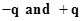are located at points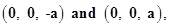respectively.

1. What is the electrostatic potential at the points?

2. Obtain the dependence of potential on the distance r of a point from the origin when r/a >> 1.

3. How much work is done in moving a small test charge from the point (5, 0, 0) to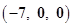along the x-axis? Does the answer change if the path of the test charge between the same points is not along the x-axis?

22:  Figure 2.34 shows a charge array known as an electric quadrupole. For a point on the axis of the quadrupole, obtain the dependence of potential on r for r/a >> 1, and contrast your results with that due to an electric dipole, and an electric monopole (i.e., a single charge).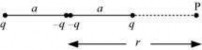23:  An electrical technician requires a capacitance of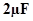in a circuit across a potential difference of 1 kV. A large number of 1 ÂµF capacitors are available to him each of which can withstand a potential difference of not more than 400 V. Suggest a possible arrangement that requires the minimum number of capacitors.

24:  What is the area of the plates of a 2 F parallel plate capacitor, given that the separation between the plates is 0.5 cm? [You will realize from your answer why ordinary capacitors are in the range of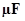or less. However, electrolytic capacitors do have a much larger capacitance (0.1 F) because of very minute separation between the conductors.]

25: Obtain the equivalent capacitance of the network in Fig. 2.35. For a 300 V supply, determine the charge and voltage across each capacitor.

26: The plates of a parallel plate capacitor have an area of 90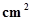each and are separated by 2.5 mm. The capacitor is charged by connecting it to a 400 V supply.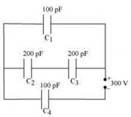1. How much electrostatic energy is stored by the capacitor?

2. View this energy as stored in the electrostatic field between the plates, and obtain the energy per unit volume u. Hence arrive at a relation between u and the magnitude of electric field E between the plates.

27:  A 4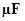capacitor is charged by a 200 V supply. It is then disconnected from the supply, and is connected to another uncharged 2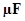capacitor. How much electrostatic energy of the first capacitor is lost in the form of heat and electromagnetic radiation?

28:  Show that the force on each plate of a parallel plate capacitor has a magnitude equal to (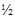) QE, where Q is the charge on the capacitor, and E is the magnitude of electric field between the plates. Explain the origin of the factor.

29: A spherical capacitor consists of two concentric spherical conductors, held in position by suitable insulating supports (Fig. 2.36).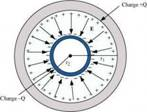Show that the capacitance of a spherical capacitor is given by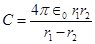where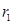and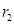are the radii of outer and inner spheres, respectively.

30:  A spherical capacitor has an inner sphere of radius 12 cm and an outer sphere of radius 13 cm. The outer sphere is earthed and the inner sphere is given a charge of 2.5 µC. The space between the concentric spheres is filled with a liquid of dielectric constant 32.

1. Determine the capacitance of the capacitor.

2. What is the potential of the inner sphere?

3. Compare the capacitance of this capacitor with that of an isolated sphere of radius 12 cm. Explain why the latter is much smaller.

1. Two large conducting spheres carrying charges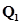and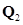are brought close to each other. Is the magnitude of electrostatic force between them exactly given by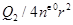, where r is the distance between their centres?

2. If Columb’s  law involved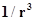dependence (instead of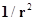), would Gauss’s  law be still true?

3. A small test charge is released at rest at a point in an electrostatic field configuration. Will it travel along the field line passing through that point?

4. What is the work done by the field of a nucleus in a complete circular orbit of the electron? What if the orbit is elliptical?

5. We know that electric field is discontinuous across the surface of a charged conductor. Is electric potential also discontinuous there?

6. What meaning would you give to the capacitance of a single conductor?

7. Guess a possible reason why water has a much greater dielectric constant (= 80) than say, mica (= 6).

32: A cylindrical capacitor has two co-axial cylinders of length 15 cm and radii 1.5 cm and1.4 cm. The outer cylinder is earthed and the inner cylinder is given a charge of 3.5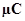. Determine the capacitance of the system and the potential of the inner cylinder. Neglect end effects (i.e., bending of field lines at the ends).

33:  A parallel plate capacitor is to be designed with a voltage rating 1 kV, using a material of dielectric constant 3 and dielectric strength about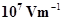. (Dielectric strength is the maximum electric field a material can tolerate without breakdown, i.e., without starting to conduct electricity through partial ionisation.) For safety, we should like the field never to exceed, say 10% of the dielectric strength. What minimum area of the plates is required to have a capacitance of 50 pF?

34:  Describe schematically the equipotential surfaces corresponding to

1. a constant electric field in the z-direction,

2. a field that uniformly increases in magnitude but remains in a constant (say, z) direction,

3. a single positive charge at the origin, and

4. a uniform grid consisting of long equally spaced parallel charged wires in a plane.

35:  In a Van de Graaff type generator a spherical metal shell is to be a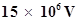electrode. The dielectric strength of the gas surrounding the electrode is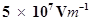. What is the minimum radius of the spherical shell required? (You will learn from this exercise why one cannot build an electrostatic generator using a very small shell which requires a small charge to acquire a high potential.)

36:  A small sphere of radius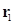and charge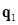is enclosed by a spherical shell of radius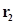and charge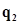. Show that ifis positive, charge will necessarily flow from the sphere to the shell (when the two are connected by a wire) no matter what the chargeon the shell is.

1. The top of the atmosphere is at about 400 kV with respect to the surface of the earth, corresponding to an electric field that decreases with altitude. Near the surface of the earth, the field is about 100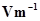. Why then do we not get an electric shock as we step out of our house into the open? (Assume the house to be a steel cage so there is no field inside!)

2. A man fixes outside his house one evening a two metre high insulating slab carrying on its top a large aluminium sheet of area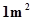. Will he get an electric shock if he touches the metal sheet next morning?

3. The discharging current in the atmosphere due to the small conductivity of air is known to be 1800 A on an average over the globe. Why then does the atmosphere not discharge itself completely in due course and become electrically neutral? In other words, what keeps the atmosphere charged?

4. What are the forms of energy into which the electrical energy of the atmosphere is dissipated during a lightning? (Hint: The earth has an electric field of about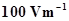at its surface in the downward direction, corresponding to a surface charge density =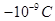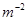. Due to the slight conductivity of the atmosphere up to about 50 km (beyond which it is good conductor), about + 1800 C is pumped every second into the earth as a whole. The earth, however, does not get discharged since thunderstorms and lightning occurring continually all over the globe pump an equal amount of negative charge on the earth.)

## NCERT Solutions for Class 12 Physics

NCERT Solutions Class 12 Physics PDF (Download) Free from myCBSEguide app and myCBSEguide website. Ncert solution class 12 physics includes text book solutions from both part 1 and part 2. NCERT Solutions for CBSE Class 12 Physics have total 20 chapters. 12 Physics NCERT Solutions in PDF for free Download on our website. Ncert physics class 12 solutions PDF and physics ncert class 12 PDF solutions with latest modifications and as per the latest CBSE syllabus are only available in myCBSEguide

To download NCERT Solutions for class 12 Physics, Chemistry, Biology, History, Political Science, Economics, Geography, Computer Science, Home Science, Accountancy, Business Studies and Home Science; do check myCBSEguide app or website. myCBSEguide provides sample papers with solution, test papers for chapter-wise practice, NCERT solutions, NCERT Exemplar solutions, quick revision notes for ready reference, CBSE guess papers and CBSE important question papers. Sample Paper all are made available through the best app for CBSE students and myCBSEguide website.### Test Generator

Create question paper PDF and online tests with your own name & logo in minutes.### myCBSEguide

Question Bank, Mock Tests, Exam Papers, NCERT Solutions, Sample Papers, Notes